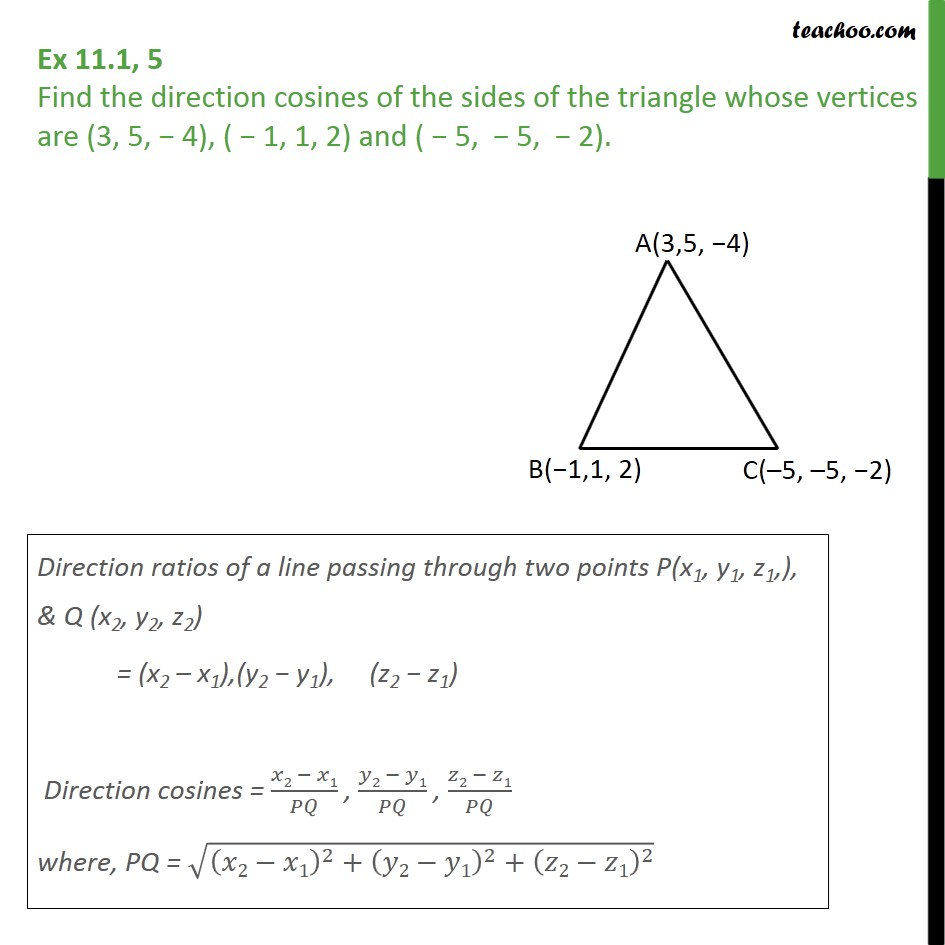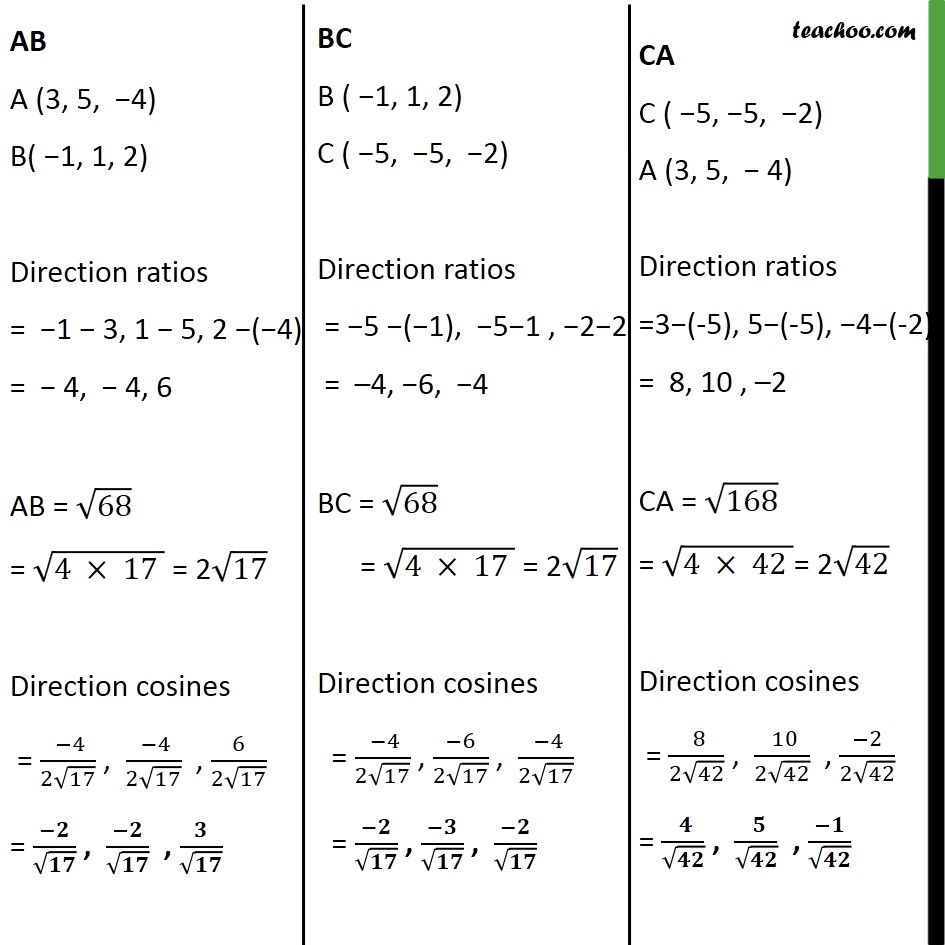1. Chapter 11 Class 12 Three Dimensional Geometry
2. Serial order wise
3. Ex 11.1

Transcript

Ex 11.1, 5 - Chapter 11 Class 12 Three Dimensional Geometry - NCERT Solution Find the direction cosines of the sides of the triangle whose vertices are (3, 5, -4), ( -1, 1, 2) and (-5, -5, -2). Direction ratios of a line passing through two points P(x1, y1, z1,), & Q (x2, y2, z2) = (x2 – x1), (y2 − y1), (z2 − z1) Direction cosines = (𝑥2 − 𝑥1)/𝑃𝑄 , (𝑦2 − 𝑦1)/𝑃𝑄 , (𝑧2 − 𝑧1)/𝑃𝑄 where, PQ = √((𝑥2−𝑥1)^2+(𝑦2−𝑦1)^2+(𝑧2−𝑧1)^2 ) AB A (3, 5, −4) B( −1, 1, 2) Direction ratios = −1 − 3, 1 − 5, 2 −(−4) = − 4, − 4, 6 AB = √68 = √(4 × 17 ) = 2√17 Direction cosines = ( −4)/(2√17) , ( −4)/(2√17) , 6/(2√17) = (−𝟐)/√𝟏𝟕 , (−𝟐)/√𝟏𝟕 , 𝟑/√𝟏𝟕 BC B ( −1, 1, 2) C ( −5, −5, −2) Direction ratios = −5 −(−1), −5−1 , −2−2 = –4, −6, −4 BC = √68 = √(4 × 17 ) = 2√17 Direction cosines = ( −4)/(2√17) , (−6)/(2√17) , ( −4)/(2√17) = (−𝟐)/√𝟏𝟕 , (−𝟑)/√𝟏𝟕 , (−𝟐)/√𝟏𝟕 CA C ( −5, −5, −2) A (3, 5, − 4) Direction ratios =3−(-5), 5−(-5), −4−(-2) = 8, 10 , –2 CA = √168 = √(4 × 42 )= 2√42 Direction cosines = ( 8)/(2√42) , ( 10)/(2√42) , (−2)/(2√42) = ( 𝟒)/√𝟒𝟐 , ( 𝟓)/√𝟒𝟐 , (−𝟏)/√𝟒𝟐

Ex 11.1